# Vector and Raster Geospatial Data

Lesson 5 with Benoît Parmentier

## Lesson Objectives

• Meet the key R packages for geospatial analysis
• Learn basic wrangling of vector and raster data
• Distinguish “scriptable” tasks from desktop GIS needs

## Specific Achievements

• Create, load and plot vector data types, or “features”
• Filter features based on associated data or on space
• Perform geometric operations on polygons
• Load, manipulate and extract raster data

Top of Section

## R Packages

The key R packages for this lesson are

### OSGeo Dependencies

In addition to the common case of depending on other R packages, these two have dependencies on system libraries.

• GDAL for read/write in geospatial data formats
• GEOS for geometry operations
• PROJ.4 for cartographic projections

System libraries cannot be installed by R’s install.packages(), but can be bundled with these packages and for private use by them. Either way, the necessary libraries are maintained by the good people at the Open Source Geospatial Foundation for free and easy distribution.

### Vector Data

The US Census website distributes county polygons (and much more) that are provided with the handouts. The sf package reads shapefiles (“.shp”) and most other vector data:

library(sf)

shp <- 'data/cb_2016_us_county_5m'
counties <- st_read(shp, stringsAsFactors = FALSE)


The counties object is a data.frame that includes a sfc, which stands for “simple feature column”. This special column is usually called “geometry” or “geom”.

> head(counties)

Simple feature collection with 6 features and 9 fields
geometry type:  MULTIPOLYGON
dimension:      XY
bbox:           xmin: -114.7556 ymin: 29.26116 xmax: -81.10192 ymax: 38.77443
epsg (SRID):    4269
STATEFP COUNTYFP COUNTYNS       AFFGEOID GEOID      NAME LSAD
1      04      015 00025445 0500000US04015 04015    Mohave   06
2      12      035 00308547 0500000US12035 12035   Flagler   06
3      20      129 00485135 0500000US20129 20129    Morton   06
4      28      093 00695770 0500000US28093 28093  Marshall   06
5      29      510 00767557 0500000US29510 29510 St. Louis   25
6      35      031 00929107 0500000US35031 35031  McKinley   06
ALAND    AWATER                       geometry
1 34475567011 387344307 MULTIPOLYGON (((-114.7556 3...
2  1257365642 221047161 MULTIPOLYGON (((-81.52366 2...
3  1889993251    507796 MULTIPOLYGON (((-102.042 37...
4  1828989833   9195190 MULTIPOLYGON (((-89.72432 3...
5   160458044  10670040 MULTIPOLYGON (((-90.31821 3...
6 14116799068  14078537 MULTIPOLYGON (((-109.0465 3...


### Geometry Types

Like any data.frame column, the geometry column is comprised of a single data type. The “MULTIPOLYGON” is just one of several standard geometric data types.

Common Types Description
POINT zero-dimensional geometry containing a single point
LINESTRING sequence of points connected by straight, non-self intersecting line pieces; one-dimensional geometry
POLYGON sequence of points in closed, non-intersecting rings; the first denotes the exterior ring, any subsequent rings denote holes
MULTI* set of * (POINT, LINESTRING, or POLYGON)

The spatial data types are built upon eachother in a logical way: lines are built from points, polygons are built from lines, and so on.

We can create any of these spatial objects from coordinates. Here’s a sfc object with a single “POINT”, corresponding to SESYNC’s postition in WGS84 degrees lat and degrees lon.

sesync <- st_sfc(st_point(
c(-76.503394, 38.976546)),
crs = st_crs(counties))


### Coordinate Reference Systems

A key feature of a geospatial data type is its associated CRS, stored as an EPSG ID and an equivalent PROJ.4 string.

> st_crs(counties)

Coordinate Reference System:
EPSG: 4269


### Bounding Box

A bounding box for all featurs in a sf data frame is generated by st_bbox().

> st_bbox(counties)

      xmin       ymin       xmax       ymax
-179.14734  -14.55255  179.77847   71.35256


The bounding box is not a static attribute—it is determined on-the-fly for the entire table or any subset of features.

library(dplyr)
counties_md <- filter(
counties,
STATEFP == '24')

> st_bbox(counties_md)

     xmin      ymin      xmax      ymax
-79.48765  37.91172 -75.04894  39.72312


### Grid

A bounding box summarizes the limits, but is not itself a geometry (not a POINT or POLYGON), even though it has a CRS attribute.

> st_crs(st_bbox(counties_md))

Coordinate Reference System:
EPSG: 4269


A rectangular grid made over a sf object is a geometry—by default, a POLYGON.

grid_md <- st_make_grid(counties_md,
n = 4)

> grid_md

Geometry set for 16 features
geometry type:  POLYGON
dimension:      XY
bbox:           xmin: -79.48765 ymin: 37.91172 xmax: -75.04894 ymax: 39.72312
epsg (SRID):    4269
First 5 geometries:

POLYGON ((-79.48765 37.91172, -78.37797 37.9117...

POLYGON ((-78.37797 37.91172, -77.26829 37.9117...

POLYGON ((-77.26829 37.91172, -76.15862 37.9117...

POLYGON ((-76.15862 37.91172, -75.04894 37.9117...

POLYGON ((-79.48765 38.36457, -78.37797 38.3645...


### Plot Layers

Spatial objects defined by sf are compatible with the plot function. Setting the plot parameter add = TRUE allows an existing plot to serve as a layer underneath the new one, so long as the CRS lines up.

plot(grid_md)
plot(counties_md['ALAND'],
plot(sesync, col = "green",
pch = 20, add = TRUE)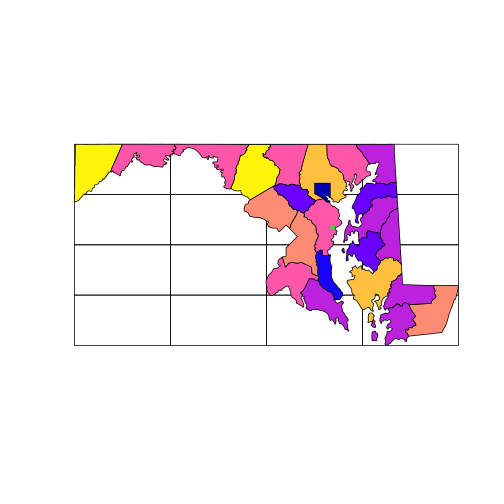But note that the plot function won’t prevent you from layering up geometries with different coordinate systems: you must safegaurd your own plots from this mistake. The arguments col and pch, by the way, are graphical parameters used in base R, see ?par.

### Spatial Subsetting

An object created with st_read is a data.frame, which is why the dplyr function filter used above on the non-geospatial column named “STATEFP” worked normally. The equivalent of a filtering operation on the “geometry” column is called a spatial “overlay”.

> st_within(sesync, counties_md)

Sparse geometry binary predicate list of length 1, where the predicate was within'
1: 5


It can be seen as a type of subsetting based on spatial (rather than numeric or string) matching. Matching is implemented with functions like st_within(x, y). The output implies that the 1^st^ (and only) point in sesync is within the 5th element of counties_md.

Question
What was the message issued by the last command all about?
It is a reminder that all geometric calculations are performed as if the coordinates (in this case longitutde and latitude) are Cartesian x,y coordinates.

The overlay functions in the sf package follow the pattern st_predicate(x, y) and perform the test “x [is] predicate y”. Some key examples are:

 st_intersects boundary or interior of x intersects boundary or interior of y st_within interior and boundary of x do not intersect exterior of y st_contains y is within x st_overlaps interior of x intersects interior of y st_equals x has the same interior and boundary as y

### Coordinate Transforms

For the next part of this lesson, we import a new polygon layer corresponding to the 1:250k map of US hydrological units (HUC) downloaded from the United States Geological Survey.

shp <- 'data/huc250k'
huc <- st_read(shp, stringsAsFactors = FALSE)


Compare the coordinate reference systems of counties and huc, as given by their Proj4 strings.

> st_crs(counties_md)$proj4string   "+proj=longlat +datum=NAD83 +no_defs"  > st_crs(huc)$proj4string

 "+proj=aea +lat_1=29.5 +lat_2=45.5 +lat_0=23 +lon_0=-96 +x_0=0 +y_0=0 +datum=NAD27 +units=m +no_defs"


The Census data uses unprojected (longitude, latitude) coordinates, but huc is in an Albers equal-area projection (indicated as “+proj=aea”).

The function st_transform() converts a sfc between coordinate reference systems, specified with the parameter crs = x. A numeric x must be a valid EPSG code; a character x is interpretted as a PROJ.4 string.

prj <- '+proj=aea +lat_1=29.5 +lat_2=45.5 \
+lat_0=23 +lon_0=-96 +x_0=0 +y_0=0 \
+ellps=GRS80 +towgs84=0,0,0,0,0,0,0 \
+units=m +no_defs'


Proj4 strings contain a reference to the type of projection, this one is another Albers Equal Area, along with numeric parameters associated with that projection. An additional important parameter that may differ between two coordinate systems is the “datum”, which indicates the standard by which the irregular surface of the Earth is approximated by an ellipsoid in the coordinates themselves.

Use st_transform() to assign the two layers to a common projection string (prj). This takes a few moments, as it requires re-calculating coordinates for every vertex of every polygon in the sfc.

counties_md <- st_transform(
counties_md,
crs = prj)
huc <- st_transform(
huc,
crs = prj)
sesync <- st_transform(
sesync,
crs = prj)

plot(counties_md$geometry) plot(huc$geometry,
border = 'blue', add = TRUE)
plot(sesync, col = 'green',
pch = 20, add = TRUE)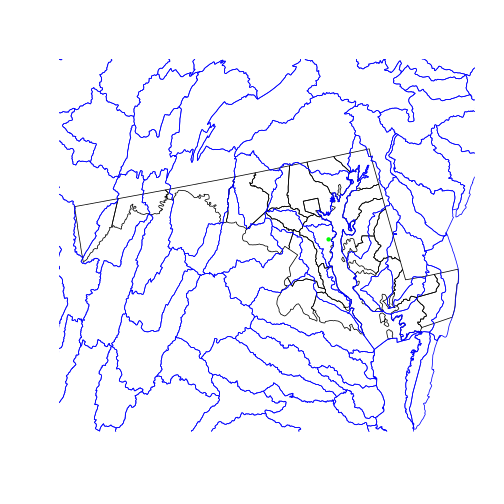### Geometric Operations

The data for a map of watershed boundaries within the state of MD is all here; in the country-wide huc and in the state boundary “surrounding” all of counties_md. To get just the huc in a MD outline:

• remove the internal county boundaries within the state
• clip the hydrological areas to their intersection with the state

The first step is a spatial union operation: we want the resulting object to combine the area covered by all the multipolygons in counties_md.

state_md <- st_union(counties_md)
plot(state_md)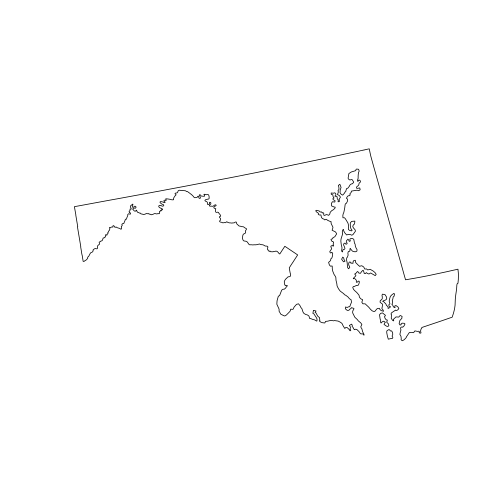To perform a union of all sub-geometries in a single sfc, we use the st_union() function with a single argument. The output, state_md, is a new sfc that is no longer a column of a data frame. Tabular data can’t safely survive a spatial union and is discarded.

The second step is a spatial intersection, since we want to limit the polygons to areas covered by both huc and state_md.

huc_md <- st_intersection(
huc,
state_md)

Warning: attribute variables are assumed to be spatially constant
throughout all geometries

plot(state_md)
plot(huc_md, border = 'blue',
col = NA, add = TRUE)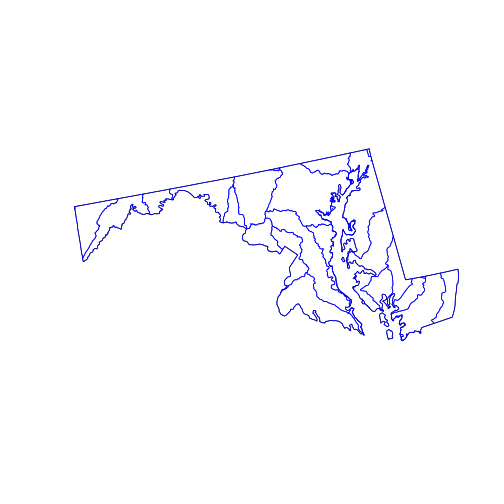The st_intersection() function intersects its first argument with the second. The individual hydrological units are preserved but any part of them (or any whole polygon) lying outside the state_md polygon is cut from the output. The attribute data remains in the corresponding records of the data.frame, but (as warned) has not been updated. For example, the “AREA” attribute of any clipped HUC does not reflect the new polygon.

The GEOS library provides many functions dealing with distances and areas. Many of these are accessible through the sf package, including:

• st_buffer: to create a buffer of specific width around a geometry
• st_distance: to calculate the shortest distance between geometries
• st_area: to calculate the area of polygons

Keep in mind that all these functions use planar geometry equations and thus become less precise over larger distances, where the Earth’s curvature is noticeable. To calculate geodesic distances that account for that curvature, checkout the geosphere package.

Top of Section

## Raster Data

Raster data is a matrix or cube with additional spatial metadata (e.g. extent, resolution, and projection) that allow its values to be mapped onto geographical space. The raster package provides the eponymous raster() function for reading the many formats of such data.

The National Land Cover Database is ‘.GRD’ format data, a lot of it. The file provided in this lesson is cropped and reduced to a lower resolution in order to speed processing.

library(raster)
nlcd <- raster("data/nlcd_agg.grd")


By default, raster data is not loaded into working memory, as you can confirm by checking the R object size with object.size(nlcd). This means that unlike most analyses in R, you can actually process raster datasets larger than the RAM available on your computer; the raster package automatically loads pieces of the data and computes on each of them in sequence.

The default print method for a raster object is a summary of metadata contained in the raster file.

> nlcd

class       : RasterLayer
dimensions  : 2514, 3004, 7552056  (nrow, ncol, ncell)
resolution  : 150, 150  (x, y)
extent      : 1394535, 1845135, 1724415, 2101515  (xmin, xmax, ymin, ymax)
coord. ref. : +proj=aea +lat_1=29.5 +lat_2=45.5 +lat_0=23 +lon_0=-96 +x_0=0 +y_0=0 +ellps=GRS80 +towgs84=0,0,0,0,0,0,0 +units=m +no_defs
data source : /nfs/public-data/training/nlcd_agg.grd
names       : nlcd_2011_landcover_2011_edition_2014_03_31
values      : 0, 95  (min, max)
attributes  :
ID      COUNT Red Green Blue Land.Cover.Class Opacity
from:   0 7854240512   0     0    0     Unclassified       1
to  : 255          0   0     0    0                        0


The plot method interprets the pixel values of the raster matrix according to a pre-defined color scheme.

> plot(nlcd)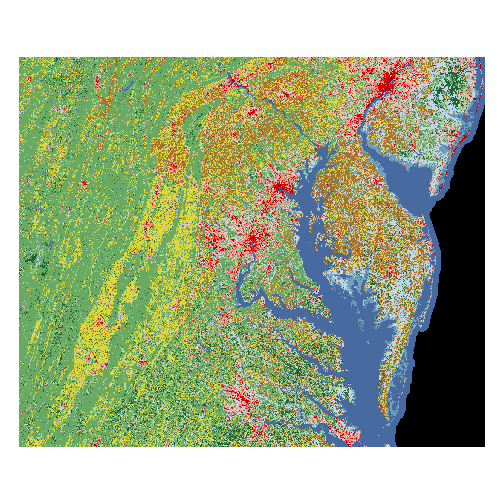The crop() function trims a raster object to a given spatial “extent” (or range of x and y values).

extent <- matrix(st_bbox(huc_md), nrow=2)
nlcd <- crop(nlcd, extent)
plot(nlcd)
plot(huc_md, col = NA, add = TRUE)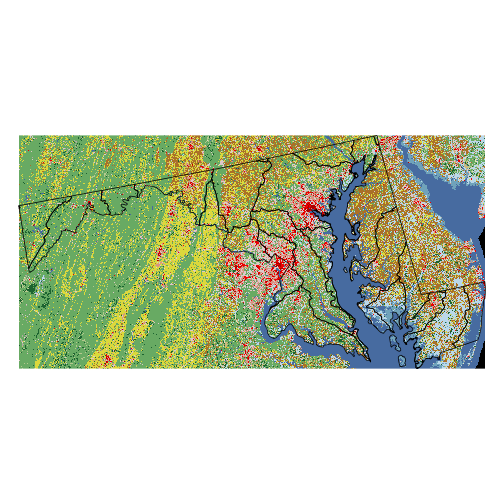The extent can be extracted from sp package objects with extent, but must be created “from scratch” for an sfc. Here, we crop the nlcd raster to the extent of the huc_md polygon, then display both layers on the same map. Also note that the transformed raster is now loaded in R memory, as indicated by the size of nlcd. We could have also saved the output to disk by specifying an optional filename argument to crop; the same is true for many other functions in the raster package.

A raster is fundamentally a data matrix, and individual pixel values can be extracted by regular matrix subscripting. For example, the value of the bottom-left corner pixel:

> nlcd[1, 1]


41


The meaning of this number is not immediately clear. For this particular dataset, the mapping of values to land cover classes is described in the data attributes:

> head(nlcd@data@attributes[])

  ID      COUNT Red     Green Blue Land.Cover.Class Opacity
1  0 7854240512   0 0.0000000    0     Unclassified       1
2  1          0   0 0.9764706    0                        1
3  2          0   0 0.0000000    0                        1
4  3          0   0 0.0000000    0                        1
5  4          0   0 0.0000000    0                        1
6  5          0   0 0.0000000    0                        1


The Land.Cover.Class vector gives string names for the land cover type corresponding to the matrix values. Note that we need to add 1 to the raster value, since these go from 0 to 255, whereas the indexing in R begins at 1.

nlcd_attr <- nlcd@data@attributes
lc_types <- nlcd_attr[]$Land.Cover.Class  > levels(lc_types)    "" "Barren Land"  "Cultivated Crops" "Deciduous Forest"  "Developed, High Intensity" "Developed, Low Intensity"  "Developed, Medium Intensity" "Developed, Open Space"  "Emergent Herbaceuous Wetlands" "Evergreen Forest"  "Hay/Pasture" "Herbaceuous"  "Mixed Forest" "Open Water"  "Perennial Snow/Ice" "Shrub/Scrub"  "Unclassified" "Woody Wetlands"  ### Raster Math Mathematical functions called on a raster gets applied to each pixel. For a single raster r, the function log(r) returns a new raster where each pixel’s value is the log of the corresponding pixel in r. Likewise, addition with r1 + r2 creates a raster where each pixel is the sum of the values from r1 and r2, and so on. Naturally, spatial attributes of rasters (e.g. extent, resolution, and projection) must match for functions that operate pixel-wise on multiple rasters. Logical operations work too: r1 > 5 returns a raster with pixel values TRUE or FALSE and is often used in combination with the mask() function. pasture <- mask(nlcd, nlcd == 81, maskvalue = FALSE) plot(pasture)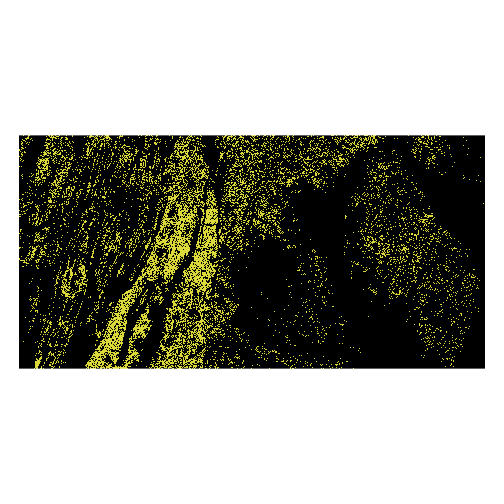A pasture raster results from unsetting pixel values where the mask (nlcd == 81) is false (maskvalue = FALSE). To further reduce the resolution of the nlcd raster, the aggregate() function combines values in a block of a given size using a given function. nlcd_agg <- aggregate(nlcd, fact = 25, fun = modal) nlcd_agg@legend <- nlcd@legend plot(nlcd_agg)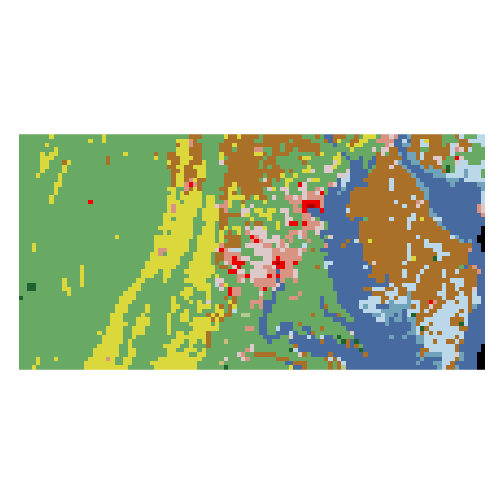Here, fact = 25 means that we are aggregating blocks 25 x 25 pixels and fun = modal indicates that the aggregate value is the mode of the original pixels (averaging would not work since land cover is a categorical variable). Top of Section ## Crossing Rasters with Vectors: Prelude Presently, to mix raster and vectors, we must convert needed sf objects to their counterpart Spatial* objects: sesync <- as(sesync, "Spatial") huc_md <- as(huc_md, "Spatial") counties_md <- as(counties_md, "Spatial")  The creation of geospatial tools in R has been a community effort, and not necessarilly a well-organized one. One current stumbling block is that the raster package, which is tightly integrated with the sp package, has not caught up to the sf package. The still-under-development stars package aims to remedy this problem and others. ## Crossing Rasters with Vectors The extract function allows subsetting and aggregation of raster values based on a vector spatial object. plot(nlcd) plot(sesync, col = 'green', pch = 16, cex = 2, add = TRUE)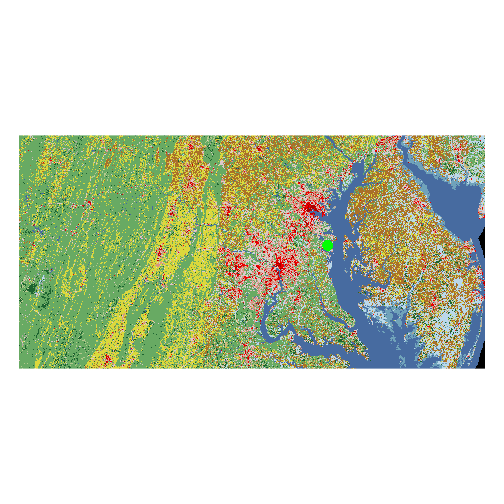When extracting by point locations (i.e. a SpatialPoints object), the result is a vector of values corresponding to each point. sesync_lc <- extract(nlcd, sesync)  > lc_types[sesync_lc + 1]   Developed, Medium Intensity 18 Levels: Barren Land Cultivated Crops ... Woody Wetlands  When extracting with a polygon, the output is a vector of all raster values for pixels falling within that polygon. county_nlcd <- extract(nlcd_agg, counties_md[1,])  > table(county_nlcd)  county_nlcd 11 21 22 23 24 41 3 1 4 5 2 1  To get a summary of raster values for each polygon in a SpatialPolygons object, add an aggregation function to extract via the fun argument. For example, fun = modal gives the most common land cover type for each polygon in huc_md. modal_lc <- extract(nlcd_agg, huc_md, fun = modal) huc_md$modal_lc <- lc_types[modal_lc + 1]

> head(huc_md)

          AREA PERIMETER HUC250K_ HUC250K_ID HUC_CODE
903 6413577966  454290.2      904        916 02050306
915 1982478663  292729.7      916        927 02040205
937 5910074657  503796.5      938        948 02070004
956 3159193443  506765.4      957        968 02060002
966 4580816836  433034.1      967        978 05020006
975 2502118608  252945.8      976        987 02070009
HUC_NAME REG  SUB    ACC      CAT         modal_lc
903     Lower Susquehanna  02 0205 020503 02050306 Deciduous Forest
915  Brandywine-Christina  02 0204 020402 02040205      Hay/Pasture
937 Conococheague-Opequon  02 0207 020700 02070004      Hay/Pasture
956     Chester-Sassafras  02 0206 020600 02060002 Cultivated Crops
966          Youghiogheny  05 0502 050200 05020006 Deciduous Forest
975              Monocacy  02 0207 020700 02070009 Cultivated Crops


Top of Section

Top of Section

## Exercises

### Exercise 1

Produce a map of Maryland counties with the county that contains SESYNC colored in red.

View solution

### Exercise 2

Use st_buffer to create a 5km buffer around the state_md border and plot it as a dotted line (plot(..., lty = 'dotted')) over the true state border. Hint: check the layer’s units with st_crs() and express any distance in those units.

View solution

### Exercise 3

The function cellStats aggregates accross an entire raster. Use it to figure out the proportion of nlcd pixels that are covered by deciduous forest (value = 41).

View solution

## Solutions

### Solution 1

> plot(counties_md\$geometry)
> overlay	<- st_within(sesync, counties_md)
> counties_sesync <- counties_md[overlay[], 'geometry']
> plot(counties_sesync, col = "red", add = TRUE)
> plot(sesync, col = 'green', pch = 20, add = TRUE)


Return

## Solution 2

> bubble_md <- st_buffer(state_md, 5000)
> plot(state_md)
> plot(bubble_md, lty = 'dotted', add = TRUE)


Return

### Solution 3

> cellStats(nlcd == 41, "mean")


Return

Top of Section

If you need to catch-up before a section of code will work, just squish it's 🍅 to copy code above it into your clipboard. Then paste into your interpreter's console, run, and you'll be ready to start in on that section. Code copied by both 🍅 and 📋 will also appear below, where you can edit first, and then copy, paste, and run again.

# Nothing here yet!`